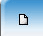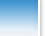Macrosystem dynamicsS.V. Konstantinov Spacecraft landing control system design based on the approximation of optimal trajectoriesCommunity informaticsEvaluation of the effectiveness of investment projectsS.V. Konstantinov Spacecraft landing control system design based on the approximation of optimal trajectoriesAbstract. The problem of general synthesis of the control system is considered. The symbolic regression method is used to solve the problem. Since the methods of this class use approaches based on heuristic algorithms, the question of determining the proximity of a solution to the optimal one remains open. In this paper, it is proposed to solve the problem of synthesis of a control system based on the approximation of the set of optimal trajectories. Initially, the optimal control problem is solved for different initial conditions, and then the resulting set of optimal trajectories is approximated by the symbolic regression method. In this case, the quality of the solution and its proximity to the optimal one is determined by the accuracy of the approximation. A computational example of solving an applied problem of synthesis of a control system for landing a spacecraft on the surface of the Moon is presented. It is experimentally shown that the found control function allows for any initial state from a given domain to obtain trajectories close to optimal in terms of the value of the quality criterion.Keywords: Optimal control, control system synthesis, symbolic regression, network operator method.PP. 3-10.DOI: 10.14357/20790279220401 References1. Boltyanskiy V.G. 1968. Matematicheskie metody optimalnogo upravleniya [Mathematical methods of optimal control]. Moscow: Nauka.2. Pontryagin L.S., Boltyanskiy V.G., Gamkrelidze R.V. and Mischenko E.F. 1983. Matematicheskaya teoriya optimalnyh processov [Mathematical theory of optimal processes]. 4-th ed. Moscow: Nauka. 392 p.3. Kurzhanski A.B. and Daryin A.N. 2019. Dynamic Programming for Impulse Feedback and Fast Controls. Springer-Verlag London Ltd.4. Krasovskiy A.A. eds. 1987. Spravochnik po teorii avtomaticheskogo upravleniya [Handbook of Automatic Control Theory]. Moscow: Nauka.  712 p.5. Kolesnikov A.A. 1994. Sinergeticheskaya teoriya upravleniya [Synergetic control theory]. Moscow: Enegroatomizdat.6. Kolesnikova S.I. 2018. Sintez sistemy upravleniya nelinejnym ob’ektom vtorogo poryadka s nepolnym opisaniem [Synthesis of a control system for a second-order nonlinearobject with an incomplete description].  Avtomatika i telemehanika [Automation and Remote Control] 9:18-30.7. Podvalny S.L. and Vasiljev E.M. 2019. Analytical Synthesis of Aggregated Regulators for Unmanned Aerial Vehicles. Journal of Mathematical Sciences 239(2):135-145.8. Kanellakopoulos I., Kokotović P. V. and Morse  A.S. 1991. Systematic design of adaptive controllers for feedback linearizable systems. IEEE Transactions on Automatic Control 36(11):1241-1253.9. Diveev A.I. 2019. Metody simvolnoj regressii dlya resheniya zadachi sinteza optimalnogo upravleniya [Symbolic regression methods for solving the optimal control synthesis problem]. Moscow: RUDN.10. Goodfellow I., Bengio Yo. and Courville A. 2016. Deep Learning. MIT Press.11. Diveev A.I. and Konstantinov S.V. 2018. Study of the practical convergence of evolutionary algorithms for the optimal program control of a wheeled robot. Journal of Computer and Systems Sciences International 57(4):561-580.12. Diveev A.I. and Sofronova E.A. 2008. Application of Network Operator Method for Synthesis of Optimal Structure and Parameters of Automatic Control System. IFAC Proceedings Volumes 41(2): 6106-6113.13. Diveev A.I. 2012. A numerical method for network operator for synthesis of a control system with uncertain initial values. Journal of Computer and Systems Sciences International 51(2):228-243.14. Liu X.-L., Duan G.-R. and Teo K.-L. 2008. Optimal soft landing control for moon lander.  Automatica 44(4):1097-1103.15. Konstantinov S.V., Khamidova U.K. and Sofronova E.A. 2019. A Novel Hybrid Method of Global Optimization Based on the Grey Wolf Optimizer and the Bees Algorithm. Procedia Computer Science 150:471-477.2023-73-2 2023-73-1 2022-72-4 2022-72-3© ФИЦ ИУ РАН 2008-2018. Создание сайта "РосИнтернет технологии".